# Thermal Study of Three-Phase Squirrel Cage Induction Motor with the Open-Phase Fault Operation Using a Lumped Parameter Network (LPTN)

Thermal Study of Three-Phase Squirrel Cage Induction Motor with the Open-Phase Fault Operation Using a Lumped Parameter Network (LPTN)
Engineering Advanced Technology Laboratory (LATAGE), Mouloud Mammeri University, BP: 15000, Tizi-Ouzou, Algeria

Corresponding Author Email:
mahdi.atig@ummto.dz
Page:
87-94
|
DOI:
https://doi.org/10.18280/ejee.230201
Received:
6 December 2019
|
Accepted:
12 March 2021
|
Published:
23 April 2021
| Citation

OPEN ACCESS

Abstract:

The aim of this paper is to estimate the induction motor temperature at both steady and transient thermal states under healthy and faulty conditions. The distribution of the temperature in the motor is calculated using thermal models based on the 2D Lumped Parameter Thermal Network (LPTN). The thermal model takes into account the heat sources, convection heat transfer and the thermal resistances in the motor. The heat flow generated by the conduction and convection in a three-phase squirrel cage induction motor is discussed. The developed model is used to study the motor thermal behavior during the opening phase situation. The results obtained by the model developed are validated by experimental tests. The tested machine is a standard three-phase, 4-pole, 2.2 kW, 380 V squirrel cage induction motor of Totally Enclosed Fan Cooled “TEFC” design manufactured in Algeria by Electro-Industries company. The simulated temperatures so obtained are in good agreement with the measured ones, and the 2D Lumped Parameter Thermal Network study seems to be appropriate to characterize the heating of the active parts of the machine under different operating conditions.

Keywords:

electric breakdown, induction motor, lumped parameter, open phase fault, thermal modelling

1. Introduction

Induction motors (IM) are robust and widely used in industrial applications because they provide good performance as well as high reliability and durability [1, 2]. However, its maximum power rating for a given frame size is governed predominantly by the temperature rise. A small temperature increase beyond the normal operating level rapidly deteriorates the properties of the insulation materials and drastically decreases the lifetime of the winding.

Induction motors (IM), can be the subject of several potential faults that affect safety, production and profitability of the installations, such as the suppression of one or more stator phases of the motor, which requires special attention. In all three-phase induction machines, when one or more phases fail, the motor breaks down or at least cannot deliver any significant torque. In the presence of the open phase fault, these machines produce more heat on both stator and rotor winding; increasing the temperature beyond the recommended limits may cause deterioration of the insulators. For this reason, the temperature must be kept below the contractual limits described in the specifications .

Over the years, several investigations of induction motor behavior under an open-phase fault condition have been presented [4-10]. The presence of the open-phase fault in induction machines creates an unbalance in the temperature distribution of the motor, which requires an important need for an accurate estimation of the temperature, particularly in those hot spots where a risk of adverse thermal conditions increases. In order to ensure a good design of electric machines, it is necessary to predict with accuracy the temperature distribution in the different sensitive parts of the machine under different conditions to prevent faults that may occur during the rotation of the motor.

Due to the presence of a certain number of heat transfers inside the machine which will have to be identified and solved, the thermal study of these electrical machines is a very complex problem. For that, two main methods are usually used: The Lumped Parameter Thermal Network (LPTN) [11-14] method and the Finite Element Analysis (FEA) [5, 12]. The disadvantage of using the finite element method is in the long computational time. However, the problem can be solved by using the Lumped Parameter Thermal Network method (LPTN). Its advantages lie in its simple mathematical form and easy implementation. Usually, a 2D Lumped Parameter Thermal Network (LPTN) is used in order to compute the motor temperature in a steady and transient state with different operating conditions. It was initially developed for the electrical network analysis, and was quickly applied successfully to thermal systems. This method simplifies the heat transfer mechanism into a thermal network with lumped parameters. The main advantage of this method is the reduced computation time taken in solving the thermal problems of electrical machines and the ease in the introduction of the convection heat transfer coefficient in natural and forced cases. Previous thermal modeling studies were often focused on the machine in normal mode, in which the main heat sources are the constant maximum copper, iron and mechanical losses [12-14].

This paper deals with the Lumped Parameter Thermal Network model (LPTN). The goal is to design a thermal model that can be used as a tool to describe the rising temperature during healthy operation and to study the effect of the open phase failure on the thermal behavior of the motor.

To check the validity of the theoretical results, an experimental investigation has been conducted on a 2.2-kW Totally Enclosed Fan-Cooled induction motor (TEFC). The calculated temperatures in steady and transient state and those obtained from measurements are compared and showed good results.

2. Thermal Model Formulation

In this paper, we are interested in the contribution of the Lumped Parameter Thermal Network (LPTN) method in the thermal modeling of the induction motor with the open phase fault. This is why we have focused on the method and not to the faults present in the machine.

Many researchers have used the Lumped Parameter Thermal Network (LPTN) for solving heat transfer problems in electrical machines [11-16]. In order to model the thermal behavior of the machine with the open phase fault, its geometry is discretized into basic elements in all three dimensions using cylindrical coordinates as shown in Figure 1. This result in the representation of the machine geometry of a set of small arc-segment elements with T-network illustration as shown in Figure 2, the corresponding thermal resistance definition is given in Eqns. (1)-(9) .

Because of the open phase fault, the heat transfer conditions in the three phases’ stator winding are different, so it is necessary to represent each of them in separate parts (phase A, phase B and phase C). Due to the different losses generated in the different phases of the machine, the temperature distribution is different. In addition, each phase of the stator is considered divided into two parts: the slot winding and end-winding, since their thermal condition is also different. Finally, according to this theory of the general arc-segment element, a whole Lumped Parameter Thermal Network (LPTN) under an open phase fault of the induction motor is developed and presented in Figure 3.

## 1.pngFigure 1. Discretization of the machine geometry for thermal analysis with 12 arc-segment elements

## 2.png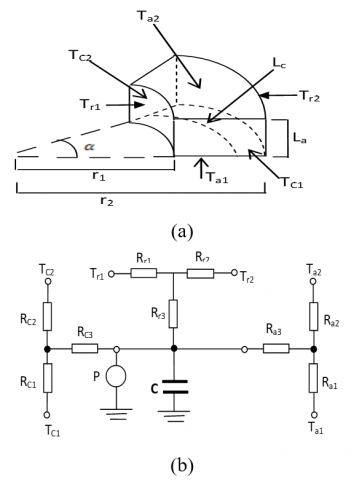Figure 2. Geometric and T-network illustration of an arc-segment element 

$R_{a 1}=R_{a 2}=\frac{180 L_{a}}{\alpha \pi \lambda_{a}\left(r_{2}^{2}-r_{1}^{2}\right)}$  (1)

$R_{a 3}=-\frac{60 L_{a}}{\alpha \pi \lambda_{a}\left(r_{2}^{2}-r_{1}^{2}\right)}$   (2)

$L_{c}=\frac{\alpha}{360} \pi\left(r_{1}-r_{2}\right)$   (3)

$R_{c 1}=R_{c 2}=\frac{L_{c}}{2 \lambda_{c} L_{a}\left(r_{2}-r_{1}\right)}$   (4)

$R_{c 3}=-\frac{L_{c}}{6 \lambda_{c} L_{a}\left(r_{2}-r_{1}\right)}$  (5)

$R_{r 1}=\frac{90}{\alpha \pi \lambda_{r} L_{a}}\left[\frac{2 r_{2}^{2} \operatorname{Ln}\left(\frac{r_{2}}{r_{1}}\right)}{\left(r_{2}^{2}-r_{1}^{2}\right)}-1\right]$  (6)

$R_{r 2}=\frac{90}{\alpha \pi \lambda_{r} L_{a}}\left[1-\frac{2 r_{1}^{2} \operatorname{Ln}\left(\frac{r_{2}}{r_{1}}\right)}{\left(r_{2}-r_{1}\right)}\right]$   (7)

$R_{r 3}=K\left[r_{2}^{2}+r_{1}^{2}-\frac{4 r_{2}^{2} r_{1}^{2} \operatorname{Ln}\left(\frac{r_{2}}{r_{1}}\right)}{\left(r_{2}^{2}-r_{1}^{2}\right)}\right]$  (8)

where:

$K=\frac{-45}{\alpha \pi \lambda_{r} L_{a}\left(r_{2}^{2}-r_{1}^{2}\right)}$  (9)

In this investigation, the circumferential heat flow has been considered only in the stator back iron and the corresponding thermal resistance’s definition is given by Eq. (4) and Eq. (5).

In order to evaluate with accuracy the magnitudes of the different resistances of the equivalent thermal network, it is necessary to know the different conductive heat transfer coefficient of the materials constituting the solid elements of the machine, and especially calculate the different convective heat exchange coefficients between the outer surface of the solid elements and the moving fluid inside the machine and the ambient. Indeed, the most important convective heat transfer coefficients to be evaluated are those between the frame and the ambient, the convective heat transfer coefficient in the end-cap air and in the air-gap. This is due to the fact that in this last location, the parts where the most losses are generated are closest together. On the other hand, for the first, the temperature rise in the different components is very sensitive to the variation of the convective heat transfer coefficient of the ambient. In our case the areas where forced convection takes place is limited to the air-gap and around the end-winding and frame to ambient.

3. Parameter Estimation and the Equivalent Thermal Circuit

3.1 Convection heat transfer coefficient in the frame

Several research papers have been published about the calculation of the heat transfer coefficient by convection between the frame and ambient of electrical machines [16-19]. Since the modeling of the convective heat transfer from the machine surface to the ambient is more challenging, the most reliable way to calculate the convection heat transfer coefficient between the ambient and the external surface of the machine is to experiment on the machine. Within literature, there exist many empirical correlations that are suited to the prediction of convection cooling from surface shapes typically seen in electrical machines. In this paper, to compute the heat transfer coefficient by convection between the frame and ambient is enough to know the total losses Ptot generated inside the machine, to measure the temperature on its external surface and to fix the temperature of the environment. Indeed, the measured convection heat transfer coefficient between the frame and ambient is given by Eq. (10):

$h=\frac{P_{t o t}}{\left(\theta_{f}-\theta_{a}\right) A_{F i n}}$  (10)

where, $\theta_{f}$ is the frame temperature (℃), $\theta_{a}$ is the ambient temperature (℃), $A_{f}=\left(A_{1}+\eta A_{f}\right)$ is the frame effective area (m2) with A1 and AFin are the surface of frame casing which is directly in contact with the cooling air and surface of the fins respectively, $\eta$ is the fin efficiency given in [16-18].

3.2 Convection heat transfer coefficient in the air-gap

It is known that the calculation of heat transfer coefficient by convection hgap (W/m2.℃) is very difficult. Because of the rotation, the air flow in the air gap is turbulent and the heat transfer problem is more complicated. The effective thermal conductivity coefficient $\lambda_{\text {eff }}$ (W/m.℃) is used and could be determined as :

$\lambda_{e f f}=0.0019 \eta^{-2.9084} R_{e}^{0.4614 . L n(3.33361 \eta)}$  (11)

where, $\eta=\frac{r_{0}}{r_{i}}$, ri and ro are the stator inner diameter and rotor outer diameter (m), respectively, $R_{e}=\frac{r_{0} \omega_{e} d}{v}$, $\omega_{e}$ is the circular velocity of the rotor (m/s), d is the length of the air gap (m) and ν is the cinematic viscosity of the air (m2/s).

3.3 Heat transfer between end-windings and end-cap air

In the end-cap region, the end-winding space presents a particular problem because the flow conditions are not clearly defined since the air is turbulent in the enclosed space and there are no smooth or flat surfaces .

Due to the forced convection in the region of the end-caps, the thermal resistance between winding and end-caps can be evaluated by the convection thermal resistances for a given surface as follows:

$R=\frac{1}{h_{C} A}$  (12)

Again, the value of hc is not simple to define. For totally enclosed machines, the value of hc can be evaluated by Eq. (13) as a function of the air speed inside the motor end-caps .

$h_{c}=6.22 v$  (13)

In addition, it can be evaluated by Eq. (14) to account for combined natural and forced convection .

$h=41.4+6.22 v$  (14)

Other similar formulas are available in the bibliography and lead to the same results [18, 21].

3.4 The equivalent thermal circuit

In this study, the machine structure has been divided into 18 elementary components as reported in Table 1:

Table 1. Components of the motor

 Components Nodes Slot winding of phase A, B and C End winding of phase A, B and C Stator yoke of phase A, B and C Stator teeth of phase A, B and C Rotor bars Yoke Air-gap End-cap air Shaft Frame 1, 2 and 3 4, 5 and 6 7, 8 and 9 10, 11 and 12 13 14 15 16 17 18

For each component, a network such as that of Figure 2(b) is carried out. Then, the total network is obtained connecting together all the single component networks, and the number of each component in Table 1, refers to the corresponding node of the total network in Figure 3. Once the total network is obtained, the motor temperature in transient state is computed as a solution of the dynamic system and expressed as follows .

$\frac{d T_{i}}{d t}=\left[C_{t}\right]^{-1}\left[P_{t}\right]-\left[C_{t}\right]^{-1}\left(\left[G_{t}\right]+\left[G_{\text {fluid }}\right]\right)\left[T_{i}\right]$  (15)

where, $C_{t}$ is the thermal capacitance matrix (J/℃), $P_{t}$ is the heat loss matrix (W), $T_{i}$ is the temperature rise matrix (℃), G is the thermal conductance matrix (W/℃) and $G_{\text {fluid }}$ is the cooling fluid matrix.

## 3.png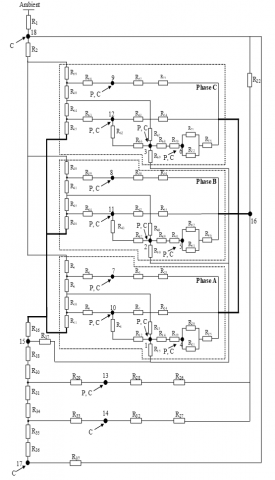Figure 3. A Lumped Parameter Thermal Network (LPTN) of a three-phase induction motor under open phase fault

Table 2. Thermal conductivities and film coefficients used in the thermal network

 Regions Thermal conductivity $\lambda_{x}(x) / \lambda_{y}(y)$ , (W/m.℃) Film coefficient  (W/m2.℃) Shaft steel Lamination Aluminium Copper Winding insulation Frame-ambient Stator iron-frame Air-gap End-cap air 40 1.98/45 204 386 0.15 - - - - - - - - - 123 467 207 27

Once the whole network obtained, the temperature rises in different elements under healthy and faulty operation are computed using Matlab/Simulink developed code, where the different heat capacities of different element of the machine are considered in the analysis of the transient operations. Table 2, gives some typical values for the thermal conductivity of solid materials and heat transfer coefficients used in the thermal investigation applied to the induction motor.

4. Experimental Investigation and Results Analysis

In order to verify the proposed Lumped Parameter Thermal network (LPTN) model, a load testing platform is established. The platform mainly includes a 2.2 kW three-phase squirrel cage induction motor, which characteristic information is shown in Table 3:

Table 3. Specification of test motor

 Parameters Values Rated power Enclosure type Frequency Rated speed Rated voltage Rated current Rated Torque Connection Insulation class Number of poles Outer diameter of stator Inner diameter of stator Air-gap length 2.2 kW TEFC 50 Hz 1420 rpm 380 V 5.2 A 15 Nm ∆ F 4 145 mm 88.5 mm 0.5 mm

In this experimental procedure we will impose two different loads: 5.4 Nm and 9.1 Nm respectively. If the motor is fed from the load of 5.4 Nm, the rms value of motor current is about 2.1 A. But, when the phase A is opened, we noted that the new rms stator currents $I_{1}, I_{2}$ and $I_{3}$ become 1.9 A, 3.35 A and 1.9 A, as shown in Figure 4 . The second test performed with a load of 9.1 Nm is the same as that of 5.4 Nm. The value of the current measured before the apparition of the defect is about 2.3 A, on the other hand the open-phase fault causes an imbalance of the currents similarly with the above test which become 2.2 A, 4.3 A and 2.2 A.

In this study, most power losses are calculated with the expressions of the balanced system. Indeed, the current obtained are used to calculate the losses in the stator winding with and without the open phase fault. Thus, their waveforms are introduced into a finite element model to determine the maximum flux density of the motor, as shown in Figure 5. Then, this maximum value was introduced into the expression of the Steinmentz equation , used to calculate core losses per unit volume in magnetic materials. The variation of B is not sinusoidal as is shown in Figure 6. A fast Fourier transform must be performed to separate the contribution of different frequency harmonics.

$B_{i}(\theta)=\sum_{i=1}^{m} B_{i, n} \cos \left(n \theta+\varphi_{i, n}\right)$  (16)

The power loss per volume unit for each harmonic is obtained for the $K_{t h}$ element from the classical expression.

$P_{i, n}=K_{h} f_{n} B_{i, n}^{\beta}+K_{e} f_{n}^{2} B_{i, n}^{2}$  (17)

where, $K_{h}$ is the hysteresis loss coefficient, $f_{n}$ is the harmonic frequency, $\beta$ is the hysteresis loss exponent, and $K_{e}$ is the eddy current loss coefficient. These values are calculated from manufacturer data sheets. The iron loss density is then computed as the sum of the density contribution of the different harmonics by:

$P_{i}=\sum P_{i, n}$   (18)

Iron losses are estimated and assigned separately for stator back iron and teeth to nodes (7, 8, 9) and (10, 11, 12) respectively. The contribution of these harmonics increases the size of these losses.

## 4a.png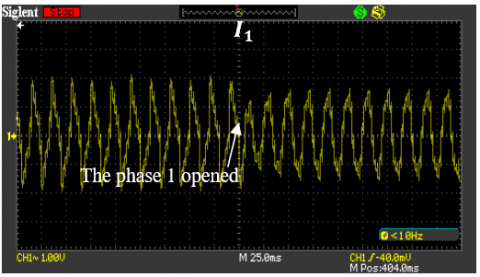(a) Current $I_{1}$  waveform

## 4b.png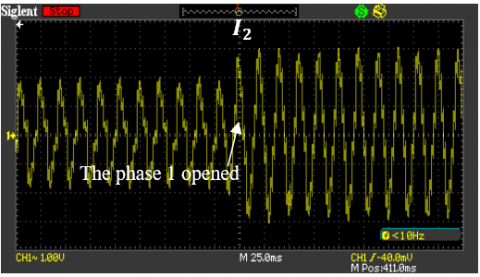(b) Current $I_{2}$  waveform

## 4c.png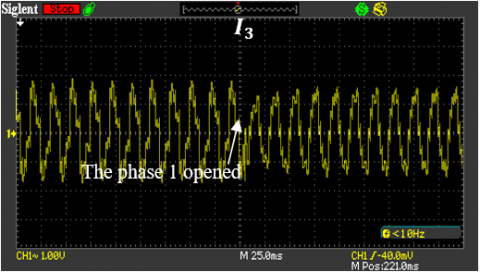(c) Current $I_{3}$  waveform

Figure 4. The waveforms of currents with a load of 5.4 Nm for healthy and faulty operating conditions 

## 5a.png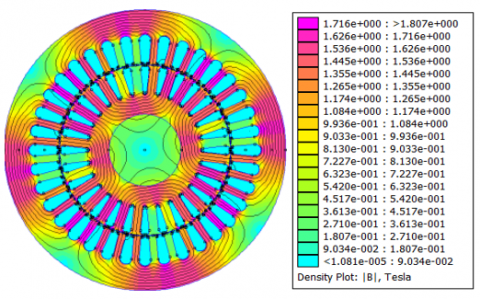(a) A load of 5.4 Nm with healthy mode

## 5b.png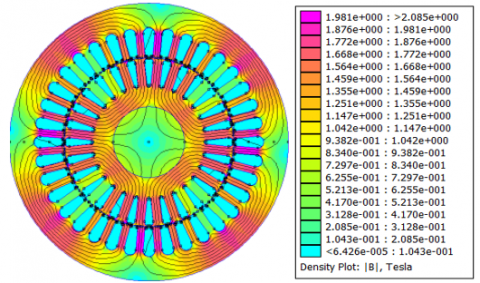(b) A load of 5.4 Nm with the open phase fault

## 5c.png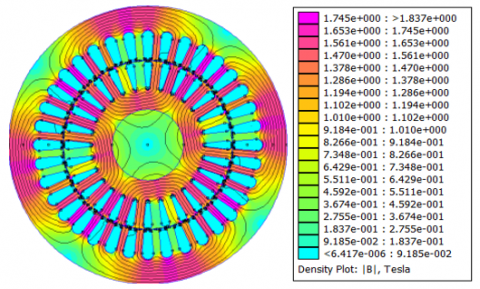(c) A load of 9.1 Nm with healthy mode

## 5d.png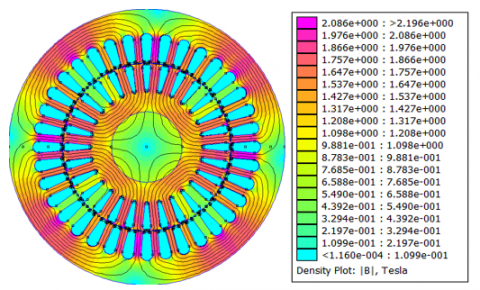(d) A load of 9.1 Nm with the open phase fault

Figure 5. Flux density distribution

## 6.jpgFigure 6. Flux density distribution in stator Back iron and its FFT

5. Results and Discussion

Table 4. Steady state temperature comparison, with a load of 5.4 Nm

 Modes Tests Frame Stator teeth Slot winding End winding cooled side Healthy Measured 38.1 50.8 52.2 52.1 mode Simulated 37.2 51.7 51 55.1 Error % 2.36 1.77 2.87 5.75 Phase 1 Measured 46.9 65.9 75.1 75.1 opened Simulated 46.6 66.1 72.8 76 Error % 0.63 0.3 3 1.19 Phase 2 Measured 46.5 68.5 65.6 65.6 opened Simulated 46.6 65.5 67.8 68.7 Error % 0.21 4.37 3.35 4.72 Phase 3 Measured 45.1 64.4 64.2 64.2 opened Simulated 46.6 65.5 67.8 68.7 Error % 3.32 1.7 5.6 7

Table 5. Steady state temperature comparison, with a load of 9.1 Nm

 Modes Tests Frame Stator teeth Slot winding End winding cooled side Healthy Measured 41.1 57.2 59.2 59.9 mode Simulated 40.6 57.4 60.3 61.7 Error % 1.21 0.34 1.85 3 Phase 1 Measured 63.6 95.3 113.5 119 opened Simulated 63.6 93.4 108.4 114.6 Error % 0 1.99 4.49 3.69 Phase 2 Measured 61.9 99.2 92.4 91.5 opened Simulated 63.6 92.4 97 98.5 Error % 2.74 6.85 4.97 7.65 Phase 3 Measured 62.5 95.2 95.2 92.3 opened Simulated 63.6 92.4 97 98.5 Error % 1.76 2.94 1.89 6.71

In Figures 7 and 8, the measured temperatures have been compared with the simulation results for healthy and faulty operations in the frame, stator teeth, slot winding and end winding cooled side, for both transient and steady state conditions. In addition, a comparison between measured and simulated temperatures at steady state is illustrated in Tables 4 and 5. Indeed, when the steady state is reached, we note that the worst discrepancy between the measured and the simulated temperatures is lower than 5.76% for healthy operation and 7.65% for faulty condition, which are acceptable in this investigation. It can be seen that the open phase defect of the induction motor results in a temperature increase up to 50% depending on the phase affected by the defect. According to the results obtained, we noted that the end winding cooled side reached a temperature of 75.1℃ with a load of 5.4 Nm and 119℃ with a load of 9.1 Nm, therefore with a rise of 43.9℃ for a difference of 3.7 Nm. Since the rated load of the induction motor is about 15 Nm with class F insulation, it is clear that the open phase at full load becomes impossible.

## 7a.png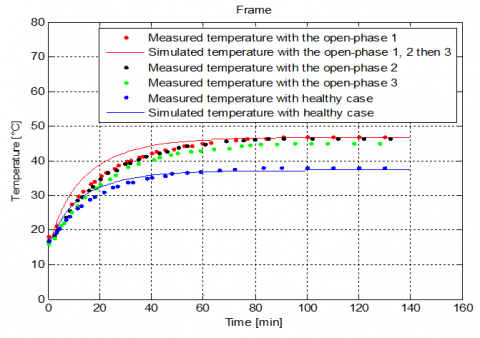## 7b.png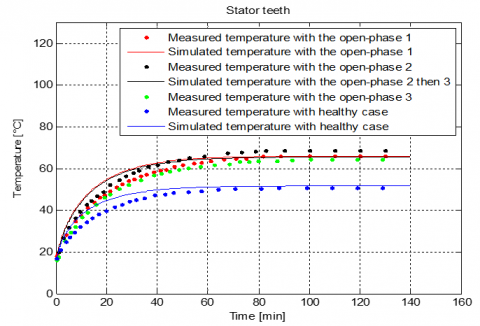## 7c.png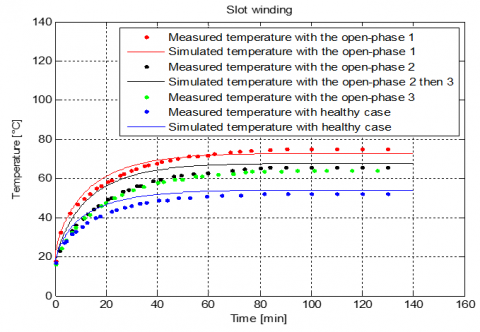## 7d.png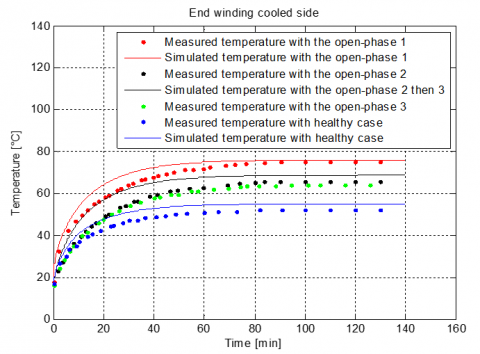Figure 7. Induction motor temperatures with a load of 5.4 Nm for healthy and faulty operations

## 8a.png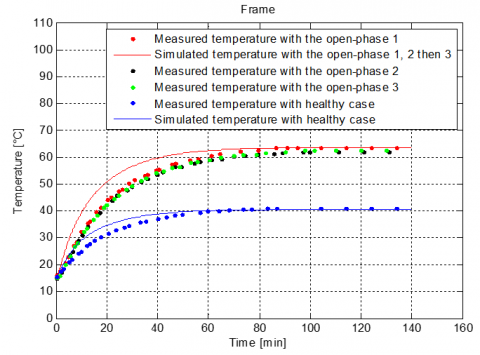## 8b.png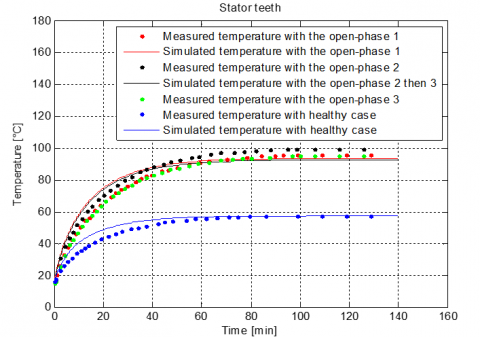## 8c.png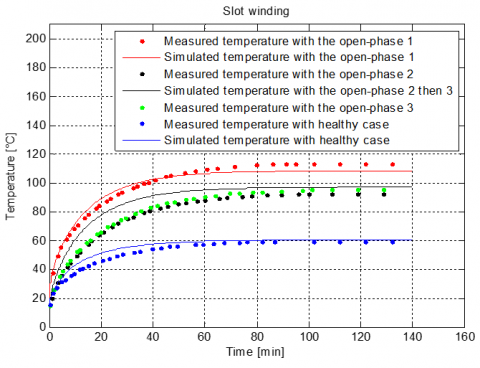## 8d.png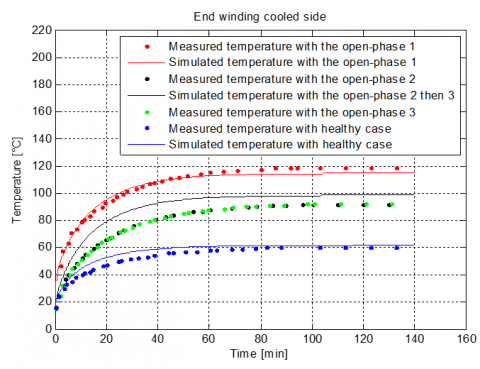Figure 8. Induction motor temperatures with a load of 9.1 Nm for healthy and faulty operations

6. Conclusions

In this paper a Lumped Parameter Thermal Network (LPTN) model for squirrel cage induction motor of Totally Enclosed Fan Cooled “TEFC” is developed and validated by measurement results at both healthy and with the open phase fault. Focusing on the thermal behavior under fault operating conditions, the electromagnetic problem gives the image of the magnetic flux distribution in full defect for two different loads as well as the evolution of the current waveforms during faults. The temperature curves for different elements show that the proposed thermal model seems to be appropriate to characterize the heating of the active parts of the machine under different operating conditions.

Although the Lumped Parameter Thermal Network (LPTN) method is very old, it has proven its efficiency and accuracy in determining the temperature in the different parts of the machine, in particular in the end winding. The 2D thermal finite element method does not take into account the end winding temperature distribution. It underestimates the actual temperature in the motor. However, the problem can be solved by using the Lumped Parameter Thermal Network (LPTN) method which gives a real picture of the temperature rise inside the machine especially in the end winding. It is useful to note that the circuit of the Figure 3, can also be used for a balanced three-phase supply with equal losses in each phase stator winding.

Acknowledgment

The authors would like to thank the members of Advanced Electrical Engineering Technology Laboratory for their invaluable help during the realization of this project.

References

 Gonzalez-Cordoba, J.L., Osornio-Rios, R.A., Granados-Lieberman, D., Romero-Troncoso, R.D.J., Valtierra-Rodriguez, M. (2017). Correlation model between voltage unbalance and mechanical overload based on thermal effect at the induction motor stator. IEEE Transactions on Energy Conversion, 32(4): 1602-1610. http://dx.doi.org/10.1109/TEC.2017.2706194

 Gnacinski, P. (2008). Effect of unbalanced voltage on windings temperature, operational life and load carrying capacity of induction machine. Energy Conversion and Management, 49(4): 761-770.http://dx.doi.org/10.1016/j.enconman.2007.07.033

 Xie, Y., Wang, Y.Y. (2014). 3D temperature field analysis of the induction motors with broken bar fault. Applied Thermal Engineering, 66(1-2): 25-34. https://doi.org/10.1016/j.applthermaleng.2014.02.008

 Guzman, H., Duran, M.J., Barrero, F., Bogado, B.,Toral, S. (2013). Speed control of five-phase induction motors with integrated open-phase fault operation using model-based predictive current control techniques. IEEE Transactions on Industrial Electronics, 61(9): 4474-4484. http://dx.doi.org/10.1109/TIE.2013.2289882

 Bianchi, N., Fornasiero, E.,Bolognani, S. (2013). Thermal analysis of a five-phase motor under faulty operations. IEEE Transactions on Industry Applications, 49(4): 1531-1538. http://dx.doi.org/10.1109/TIA.2013.2258452

 Hajary, A., Kianinezhad, R., Seifossadat, S.G., Mortazavi, S.S., Saffarian, A. (2019). Detection and localization of open-phase fault in three-phase induction motor drives using second order rotational park transformation. IEEE Transactions on Power Electronics, 34(11): 11241-11252. http://dx.doi.org/10.1109/TPEL.2019.2901598

 Strankowski, P., Jąderko, A., Blecharz, K., Morawiec, M. (2019). Fault detection algorithm for five-phase induction motor drive. In 2019 Applications of Electromagnetics in Modern Engineering and Medicine (PTZE), Janow Podlaski, Poland, pp. 215-218. http://dx.doi.org/10.23919/PTZE.2019.8781715

 Duran, M.J., Gonzalez-Prieto, I., Rios-Garcia, N., Barrero, F. (2017). A simple, fast, and robust open-phase fault detection technique for six-phase induction motor drives. IEEE Transactions on Power Electronics, 33(1): 547-557. http://dx.doi.org/10.1109/TPEL.2017.2670924

 Bermudez, M., Gonzalez-Prieto, I., Barrero, F., Guzman, H., Duran, M.J., Kestelyn, X. (2016). Open-phase fault-tolerant direct torque control technique for five-phase induction motor drives. IEEE Transactions on Industrial Electronics, 64(2): 902-911. http://dx.doi.org/10.1109/TIE.2016.2610941

 de Souza, T.S., Bastos, R.R., Cardoso Filho, B.J. (2018). Modeling and control of a nine-phase induction machine with open phases. IEEE Transactions on Industry Applications, 54(6): 6576-6585. http://dx.doi.org/10.1109/TIA.2018.2852282

 Mezani, S., Takorabet, N., Laporte, B. (2005). A combined electromagnetic and thermal analysis of induction motors. IEEE Transactions on Magnetics, 41(5): 1572-1575. http://dx.doi.org/10.1109/TMAG.2005.845044

 Bouheraoua, M., Benamrouche, N., Bousbaine, A. (2011). A more refined thermal model for a totally enclosed fan-cooled induction motor. Electric Power Components and systems, 40(2): 179-194. http://dx.doi.org/10.1080/15325008.2011.629334

 Mellor, P.H., Roberts, D., Turner, D.R. (1991). Lumped parameter thermal model for electrical machines of TEFC design. In IEE Proceedings B (Electric Power Applications), 138(5): 205-218. http://dx.doi.org/10.1049/ip-b.1991.0025

 Sciascera, C., Giangrande, P., Papini, L., Gerada, C., Galea, M. (2017). Analytical thermal model for fast stator winding temperature prediction. IEEE Transactions on Industrial Electronics, 64(8): 6116-6126. http://dx.doi.org/10.1109/TIE.2017.2682010

 Nair, D.G., Rasilo, P., Arkkio, A. (2018). Sensitivity analysis of inverse thermal modeling to determine power losses in electrical machines. IEEE Transactions on Magnetics, 54(11): 1-5. http://dx.doi.org/10.1109/TMAG.2018.2853084

 Bousbaine, A. (1993). An investigation into the thermal modelling of induction motors. Doctoral dissertation, University of Sheffield.

 Simpson, N., Wrobel, R., Mellor, P.H. (2014). A general arc-segment element for three-dimensional thermal modeling. IEEE Transactions on Magnetics, 50(2): 265-268. http://dx.doi.org/10.1109/TMAG.2013.2278866

 Rohsenow, W.M., Hartnett, J.P., Ganic, E.N., Richardson, P.D. (1986). Handbook of heat transfer fundamentals. J. Appl. Mech. Mar, 53(1): 232-233. https://doi.org/10.1115/1.3171729

 Valenzuela, M.A., Tapia, J.A. (2008). Heat transfer and thermal design of finned frames for TEFC variable-speed motors. IEEE Transactions on Industrial Electronics, 55(10): 3500-3508. http://dx.doi.org/10.1109/TIE.2008.928150

 Boglietti, A., Cavagnino, A. (2006). Analysis of the endwinding cooling effects in TEFC induction motors. In Conference Record of the 2006 IEEE Industry Applications Conference Forty-First IAS Annual Meeting, Tampa, FL, USA, pp. 797-804. http://dx.doi.org/10.1109/IAS.2006.256617

 Boglietti, A., Cavagnino, A., Popescu, M., Staton, D. (2013). Thermal model and analysis of wound-rotor induction machine. IEEE Transactions on Industry Applications, 49(5): 2078-2085.http://dx.doi.org/10.1109/TIA.2013.2261444

 Atig, M., Bouheraoua, M., Fekik, A. (2018). An experimental investigation of heating in induction motor under open phase fault. International Journal of Electrical & Computer Engineering, 8(3). http://doi.org/10.11591/ijece.v8i3.pp1288-1296

 Steinmetz, C.P. (1892). On the law of hysteresis. Transactions of the American Institute of Electrical Engineers, IX(1): 1-64. http://dx.doi.org/10.1109/T-AIEE.1892.5570437